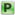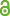Hauptmenü
• Autor
• Kimeswenger, Arno
• Steinbach, Olaf
• Unger, Gerhard
• TitelCoupled finite and boundary element methods for fluid-solid interaction eigenvalue problems
• Datei
• DOI10.1137/13093755x
• Persistent Identifier
• Erschienen inSIAM Journal on Numerical Analysis
• Band52
• Erscheinungsjahr2014
• Heft5
• Seiten2400-2414
• LicenceCC BY
• ISSN1095-7170
• Zugriffsrechte• Download Statistik589
• Peer ReviewJa
• AbstractWe analyze the approximation of a vibro-acoustic eigenvalue problem for an elas- tic body which is submerged in a compressible inviscid fluid in ℝ³. As model the time-harmonic elastodynamic and the Helmholtz equation are used and are coupled in a strong sense via the standard transmission conditions on the interface between the solid and the fluid. Our approach is based on a coupling of the field equations for the solid with boundary integral equations for the fluid. The coupled formulation of the eigenvalue problem leads to a nonlinear eigenvalue problem with respect to the eigenvalue parameter since the frequency occurs nonlinearly in the used bound- ary integral operators for the Helmholtz equation. The nonlinear eigenvalue problem and its Galerkin discretization are analyzed within the framework of eigenvalue prob- lems for Fredholm operator-valued functions where convergence is shown and error estimates are given. For the numerical solution of the discretized nonlinear matrix eigenvalue problem the contour integral method is a reliable method which is demon- strated by some numerical examples.